# Affine geometry

In mathematics, affine geometry is what remains of Euclidean geometry when ignoring (mathematicians often say "forgetting") the metric notions of distance and angle. As the notion of parallel lines is one of the main properties that is independent of any metric, affine geometry is often considered as the study of parallel lines. Therefore, Playfair's axiom (Given a line L and a point P not on L, there is exactly one line parallel to L that passes through P.) is fundamental in affine geometry. Comparisons of figures in affine geometry are made with affine transformations, which are mappings that preserve alignment of points and parallelism of lines. Affine geometry can be developed in two ways that are essentially equivalent. In synthetic geometry, an affine space is a set of points to which is associated a set of lines, which satisfy some axioms (such as Playfair's axiom). Affine geometry can also be developed on the basis of linear algebra. In this context an affine space is a set of points equipped with a set of transformations (that is bijective mappings), the translations, which forms a vector space (over a given field, commonly the real numbers), and such that for any given ordered pair of points there is a unique translation sending the first point to the second; the composition of two translations is their sum in the vector space of the translations. In more concrete terms, this amounts to having an operation that associates to any ordered pair of points a vector and another operation that allows translation of a point by a vector to give another point; these operations are required to satisfy a number of axioms (notably that two successive translations have the effect of translation by the sum vector). By choosing any point as "origin", the points are in one-to-one correspondence with the vectors, but there is no preferred choice for the origin; thus an affine space may be viewed as obtained from its associated vector space by "forgetting" the origin (zero vector). The idea of forgetting the metric can be applied in the theory of manifolds. That is developed in the article on the affine connection. (Wikipedia).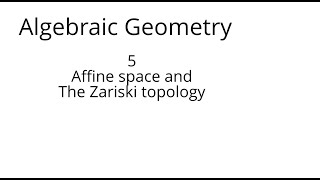algebraic geometry 5 Affine space and the Zariski topology

This lecture is part of an online algebraic geometry course, based on chapter I of "Algebraic geometry" by Hartshorne. It covers the definition of affine space and its Zariski topology.

From playlist Algebraic geometry I: Varieties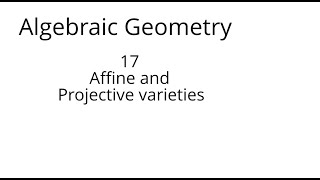algebraic geometry 17 Affine and projective varieties

This lecture is part of an online algebraic geometry course, based on chapter I of "Algebraic geometry" by Hartshorne. It covers the relation between affine and projective varieties, with some examples such as a cubic curve and the twisted cubic.

From playlist Algebraic geometry I: Varieties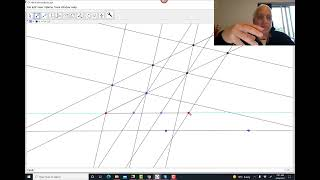Calculus and affine geometry of the magical parabola | Algebraic Calc and dCB curves 3 | Wild Egg

Algebraic Calculus naturally lives in affine geometry, not Euclidean geometry. Affine geometry is the geometry of parallelism, or (almost the same thing) --- the geometry of pure linear algebra. The parabola is characterized projectively in this geometry as the unique conic which is tangen

From playlist Algebraic Calculus One Info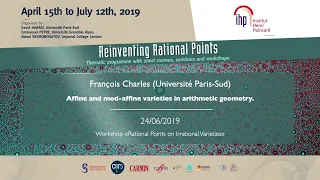Affine and mod-affine varieties in arithmetic geometry. - Charles - Workshop 2 - CEB T2 2019

François Charles (Université Paris-Sud) / 24.06.2019 Affine and mod-affine varieties in arithmetic geometry. We will explain how studying arithmetic versions of affine schemes and their bira- tional modifications leads to a generalization to arbitrary schemes of both Fekete’s theorem on

From playlist 2019 - T2 - Reinventing rational points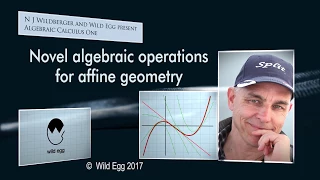Novel Algebraic Operations for Affine Geometry | Algebraic Calculus One | Wild Egg

We introduce some novel conventions to help us set up the foundations of affine geometry. We learn about differences of points, sums of points and vectors, affine combinations and vector proportions. And then use these to state a number of important results from affine geometry, including

From playlist Algebraic Calculus One from Wild Egg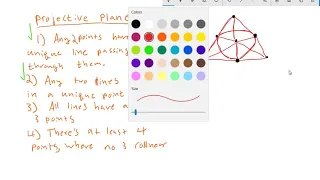Introduction to Projective Geometry (Part 2)

The second video in a series about projective geometry. We list the axioms for projective planes, give an examle of a projective plane with finitely many points, and define the real projective plane.

From playlist Introduction to Projective Geometry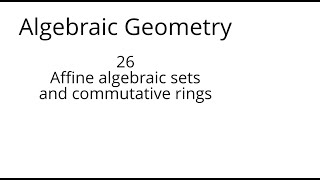algebraic geometry 26 Affine algebraic sets and commutative rings

This lecture is part of an online algebraic geometry course, based on chapter I of "Algebraic geometry" by Hartshorne. It covers the relation between morphisms of affine algebraic sets and homomorphisms of commutative rings. As examples it describes some homomorphisms of commutative rings

From playlist Algebraic geometry I: Varieties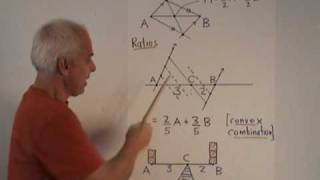Affine geometry and barycentric coordinates | WildTrig: Intro to Rational Trigonometry

Affine geometry is the geometry of parallel lines. Using parallelism, we show how to construct a ruled line, how to find the midpoint of a segment, and divide a segment into a given ratio. We connect this to Archimedes law of the lever, and then extend to barycentric coordinates with respe

From playlist WildTrig: Intro to Rational Trigonometry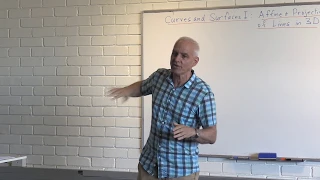CurvesSurfaces1: Affine and Projective Geometry, and the Problem of Lines

N J Wildberger from UNSW introduces a new series on Curves and Surfaces, aiming for a concrete and more geometrical approach to Algebraic Geometry. This introductory lecture introduces basic notation and terminology for affine and projective points, and associated vector spaces, using proj

From playlist MathSeminars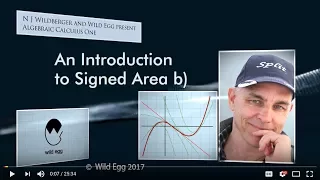Introduction to Signed Area b) | Algebraic Calculus One | Wild Egg

This is a lecture in the Algebraic Calculus One course, which will present an exciting new approach to calculus, sticking with rational numbers and high school algebra, and avoiding all "infinite processes", "real numbers" and other modern fantasies. The course will be carefully framed on

From playlist Algebraic Calculus One from Wild Egg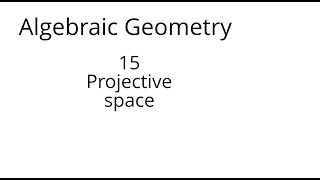algebraic geometry 15 Projective space

This lecture is part of an online algebraic geometry course, based on chapter I of "Algebraic geometry" by Hartshorne. It introduces projective space and describes the synthetic and analytic approaches to projective geometry

From playlist Algebraic geometry I: Varietiesalgebraic geometry 29 Automorphisms of space

This lecture is part of an online algebraic geometry course, based on chapter I of "Algebraic geometry" by Hartshorne. It describes the automorphisms of affine and projective space, and gives a brief discussion of the Jacobian conjecture.

From playlist Algebraic geometry I: Varieties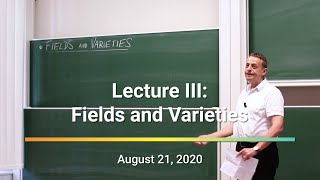Tropical Geometry - Lecture 3 - Fields and Varieties | Bernd Sturmfels

Twelve lectures on Tropical Geometry by Bernd Sturmfels (Max Planck Institute for Mathematics in the Sciences | Leipzig, Germany) We recommend supplementing these lectures by reading the book "Introduction to Tropical Geometry" (Maclagan, Sturmfels - 2015 - American Mathematical Society)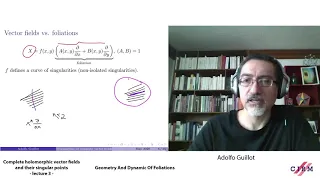Adolfo Guillot: Complete holomorphic vector fields and their singular points - lecture 3

CIRM VIRTUAL EVENT Recorded during the research school "Geometry and Dynamics of Foliations " the May 11, 2020 by the Centre International de Rencontres Mathématiques (Marseille, France) Filmmaker: Guillaume Hennenfent Find this video and other talks given by worldwide mathematicians on C

From playlist Virtual Conference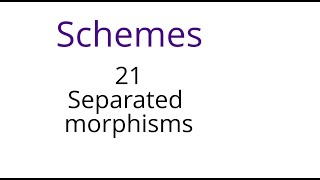Schemes 21: Separated morphisms

This lecture is part of an online algebraic geometry course on schemes, based on chapter II of "Algebraic geometry" by Hartshorne.. We define separated and quasi-separated schemes and morphisms, give a few examples, and show that if a scheme has a separated morphism to an affine scheme the

From playlist Algebraic geometry II: Schemes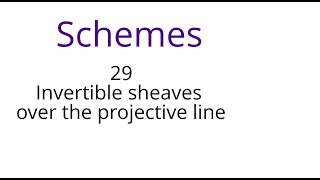Schemes 29: Invertible sheaves over the projective line

This lecture is part of an online algebraic geometry course on schemes, based on chapter II of "Algebraic geometry" by Hartshorne. In this lecture we classify the invertible sheaves over the projective line, and use them to show that several properties of quasiprojective sheaves over affi

From playlist Algebraic geometry II: Schemes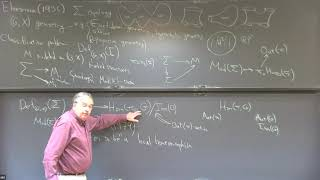Dynamics on character varieties - William Goldman

Character Varieties, Dynamics and Arithmetic Topic: Dynamics on character varieties Speaker: William Goldman Affiliation: University of Maryland; Member, School of Mathematics Date: November 17, 2021 In these two talks, I will describe how the classification of locally homogeneous geomet

From playlist Mathematics